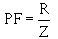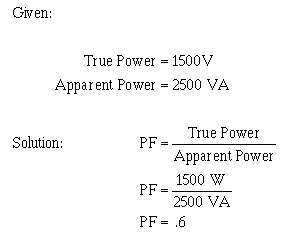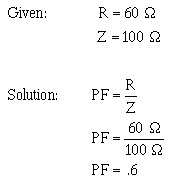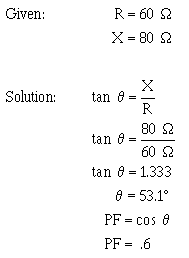Power FactorCustom SearchPower Factor The POWER FACTOR is a number (represented as a decimal or a percentage) that represents the portion of the apparent power dissipated in a circuit. If you are familiar with trigonometry, the easiest way to find the power factor is to find the cosine of the phase angle q. The cosine of the phase angle is equal to the power factor. You do not need to use trigonometry to find the power factor. Since the power dissipated in a circuit is true power, then:If true power and apparent power are known you can use the formula shown above. Going one step further, another formula for power factor can be developed. By substituting the equations for true power and apparent power in the formula for power factor, you get:Since current in a series circuit is the same in all parts of the circuit, IRequals IZ. Therefore, in a series circuit,For example, to compute the power factor for the series circuit shown in figure 4-10, any of the above methods may be used.Another method:If you are familiar with trigonometry you can use it to solve for angleq and the power factor by referring to the tables in appendices V and VI.NOTE: As stated earlier the power factor can be expressed as a decimal or percentage. In this example the decimal number .6 could also be expressed as 60%. Q.28 What is the power factor of a circuit? Q.29 What is a general formula used to calculate the power factor of a circuit?Integrated Publishing, Inc. - A (SDVOSB) Service Disabled Veteran Owned Small Business